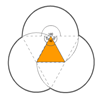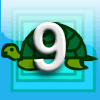Search by Topic

Resources tagged with Angle properties of polygons similar to All about Ratios:

Filter by: Content type:
Age range:
Challenge level:

There are 22 results

Broad Topics > Angles, Polygons, and Geometrical Proof > Angle properties of polygonsA Sameness Surely

Age 14 to 16 Challenge Level:

Triangle ABC has a right angle at C. ACRS and CBPQ are squares. ST and PU are perpendicular to AB produced. Show that ST + PU = ABMaking Sixty

Age 14 to 16 Challenge Level:

Why does this fold create an angle of sixty degrees?Golden Triangle

Age 16 to 18 Challenge Level:

Three triangles ABC, CBD and ABD (where D is a point on AC) are all isosceles. Find all the angles. Prove that the ratio of AB to BC is equal to the golden ratio.Pentakite

Age 14 to 18 Challenge Level:

ABCDE is a regular pentagon of side length one unit. BC produced meets ED produced at F. Show that triangle CDF is congruent to triangle EDB. Find the length of BE.Pent

Age 14 to 18 Challenge Level:

The diagram shows a regular pentagon with sides of unit length. Find all the angles in the diagram. Prove that the quadrilateral shown in red is a rhombus.Logo Challenge 3 - Star Square

Age 7 to 16 Challenge Level:

Creating designs with squares - using the REPEAT command in LOGO. This requires some careful thought on anglesLOGO Challenge 4 - Squares to Procedures

Age 11 to 16 Challenge Level:

This LOGO Challenge emphasises the idea of breaking down a problem into smaller manageable parts. Working on squares and angles.At a Glance

Age 14 to 16 Challenge Level:

The area of a regular pentagon looks about twice as a big as the pentangle star drawn within it. Is it?Angles in Three Squares

Age 11 to 16 Challenge Level:

Drawing the right diagram can help you to prove a result about the angles in a line of squares.Triangles and Petals

Age 14 to 16 Challenge Level:

An equilateral triangle rotates around regular polygons and produces an outline like a flower. What are the perimeters of the different flowers?Darts and Kites

Age 14 to 16 Challenge Level:

Explore the geometry of these dart and kite shapes!Arclets Explained

Age 11 to 16

This article gives an wonderful insight into students working on the Arclets problem that first appeared in the Sept 2002 edition of the NRICH website.Dodecawhat

Age 14 to 16 Challenge Level:

Follow instructions to fold sheets of A4 paper into pentagons and assemble them to form a dodecahedron. Calculate the error in the angle of the not perfectly regular pentagons you make.Age 14 to 16 Challenge Level:

Make five different quadrilaterals on a nine-point pegboard, without using the centre peg. Work out the angles in each quadrilateral you make. Now, what other relationships you can see?Tricircle

Age 14 to 16 Challenge Level:

The centre of the larger circle is at the midpoint of one side of an equilateral triangle and the circle touches the other two sides of the triangle. A smaller circle touches the larger circle and. . . .First Forward Into Logo 7: Angles of Polygons

Age 11 to 18 Challenge Level:

More Logo for beginners. Learn to calculate exterior angles and draw regular polygons using procedures and variables.Age 16 to 18 Short Challenge Level:

Can you work out where the blue-and-red brick roads end?Semi-regular Tessellations

Age 11 to 16 Challenge Level:

Semi-regular tessellations combine two or more different regular polygons to fill the plane. Can you find all the semi-regular tessellations?First Forward Into Logo 9: Stars

Age 11 to 18 Challenge Level:

Turn through bigger angles and draw stars with Logo.No Right Angle Here

Age 14 to 16 Challenge Level:

Prove that the internal angle bisectors of a triangle will never be perpendicular to each other.Terminology

Age 14 to 16 Challenge Level:

Given an equilateral triangle inside an isosceles triangle, can you find a relationship between the angles?